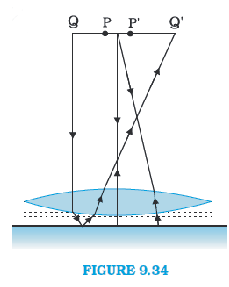# Q 9.32  Figure 9.34 shows an equiconvex lens (of refractive index 1.50) in contact with a liquid layer on top of a   plane mirror. A small needle with its tip on the principal axis is moved along the axis until its inverted image is found at the position of the needle. The distance of the needle from the lens is measured to be 45.0cm. The liquid is removed and the experiment is repeated. The new distance is measured to be 30.0cm. What is the refractive index of the liquid?P Pankaj Sanodiya

Given

The focal length of the convex lens

here liquid is acting like the mirror so,

the focal length of the liquid

the focal length of the system(convex + liquid)

Equivalent focal length when two optical systems are in contact

Now, let us assume refractive index of the lens be

The radius of curvature are  and .

As we know,

Now, let refractive index of liquid be

The radius of curvature of liquid in plane mirror side = infinite

Radius of curvature of liquid in lens side R  = -30cm

As we know,

Therefore the refractive index of the liquid is 1.33.

Exams
Articles
Questions